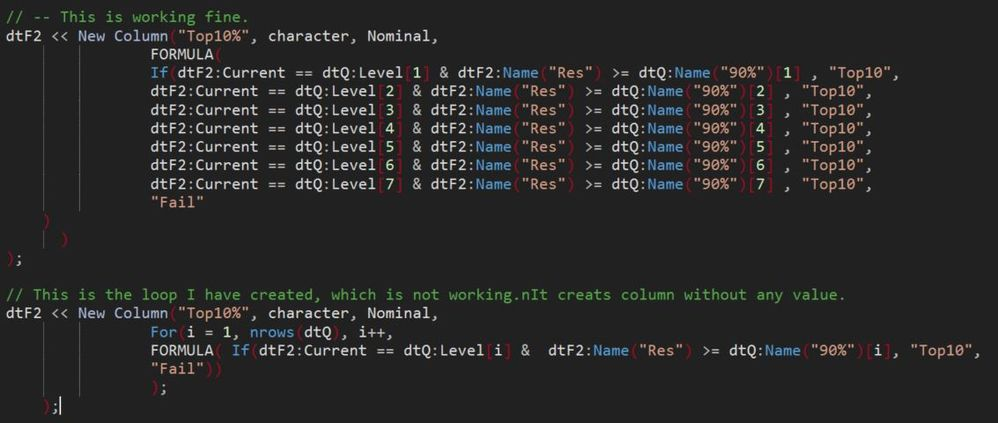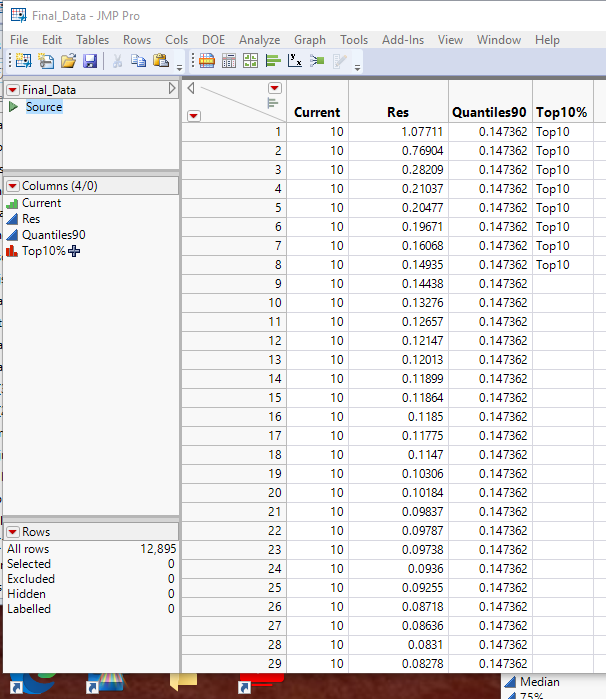Our World Statistics Day conversations have been a great reminder of how much statistics can inform our lives. Do you have an example of how statistics has made a difference in your life? Share your story with the Community!
Choose Language Hide Translation Bar
Highlighted

## Issue in JMP script for loop

Hello, I have two questions -

Ques1 --

I have a simple data (attached) and a script working fine but as long as I want convert it into a for loop. Not getting the right thing.

AIM - I want the top10% resistance value for each current. My data is not symmetric, means number of data points for each current is different.

In the same data there are only 7 current values and in real data, it can take 100s of values.

Approach -- I am generating a Quantile table and then comparing my resistance value by 90% quantile value and adding an extra column 'Top10%'. Everything is working fine for sample data with 7 current values, but I need a loop for it. Can super users point me what is the mistake I am doing in my for loop, this will help to learn how implement for loops.?

Any help ?Ques2 --What is the best way to get top 95% data ?

Any help here ?

1 ACCEPTED SOLUTION

Accepted Solutions
Highlighted

## Re: Issue in JMP script for loop

I believe the below script is a simpler methodology to get to the results you want``````Names Default To Here( 1 );
dtF2 = Data Table( "Final_Data" );

dtSum = dtF2 << Summary(
invisible,
Group( :Current ),
Quantiles( 90, :Res ),
Freq( "None" ),
Weight( "None" ),
statistics column name format( "stat" ),
link to original data table( 0 )
);

dtSum << delete columns( N Rows );

dtF2 << Update(
With( dtSum ),
Match Columns( :Current = :Current )
);

Close( dtSum, nosave );

dtF2 << New Column( "Top10%",
character,
formula( If( :Res >= :Quantiles90, "Top10" ) )
);``````

Jim
4 REPLIES 4
Highlighted

## Re: Issue in JMP script for loop

I believe the below script is a simpler methodology to get to the results you want``````Names Default To Here( 1 );
dtF2 = Data Table( "Final_Data" );

dtSum = dtF2 << Summary(
invisible,
Group( :Current ),
Quantiles( 90, :Res ),
Freq( "None" ),
Weight( "None" ),
statistics column name format( "stat" ),
link to original data table( 0 )
);

dtSum << delete columns( N Rows );

dtF2 << Update(
With( dtSum ),
Match Columns( :Current = :Current )
);

Close( dtSum, nosave );

dtF2 << New Column( "Top10%",
character,
formula( If( :Res >= :Quantiles90, "Top10" ) )
);``````

Jim
Highlighted

## Re: Issue in JMP script for loop

Yes, perfectly fine. Much better and simpler way to do it. I was not aware that we can get quantile as well from summary table. I am implementing it the way you described.

Apart from the quantile and top 10% data, Any comment what was wrong in my "for loop" ?

Thanks for the help.

Highlighted

## Re: Issue in JMP script for loop

1. A column can only have 1 Formula.  Having the Formula definition within the For() loop would specify a different formula for each loop.  I don't know exactly what JMP would do, at best, it would replace each formula when a new one was created, ending up with just the last one created.
2. By setting the "Fail" value within each If, the only time your logic would be accurate, is if the Last Level is the level for the current row.  Otherwise, let's say, Level 3 is the current level for the given row.  JMP would set the value in the If statement for Level 1 to Fail, Level 2, to Fail, Level 3 to Top10%, but the For() Loop continues, so then testing for Level 4, will result in Fail.........and all the rest of the levels.
3. With a formula for a column that has variable values defined outside of the formula (i.e. dtF2, dtQ and the references to the columns in the dtQ data table), those values and the data table will have to be present whenever the data table with the formula is opened up.  So what you need to do, when using such formulas, is to delete the formula, once the data table has run the formula.
``dtF2:Name("Top10%") << delete formula;``

Here is a version of the formula that I think would work.....I haven't tested it, but I think you will see the approach.

``````dtF2 << New Column( "Top10%",
character,

FORMULA(
For( i = 1, N Rows( dtQ ), i++,
x = "";
If( dtF2:Current == dtQ:Level[i] & dtF2:Name( "Res" ) >= dtQ:Name( "90%" )[i],
x = "Top10"
);
);
If( x == "", x = "Fail" );
x;
)
);``````
Jim
Highlighted

## Re: Issue in JMP script for loop

Thanks for clear and nice explanation. Very helpful to learn how  Column Formulas works. Thanks again.

Article Labels